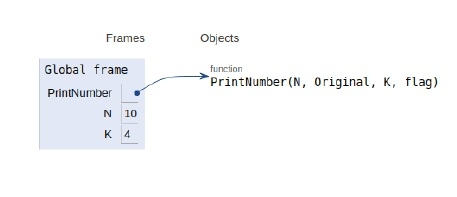# Python Program for Print Number series without using any loop

Problem statement − Given Two number N and K, our problem is to subtract a number K from N until number(N) is greater than zero(0), once the N becomes negative or zero then we start adding K to it until that number become the original number(N).

For example,

N = 10
K = 4
Output will be: 10 6 2 -2 2 6 10

## Algorithm

1. we call the function again and again until N is greater than zero (in every function
call we subtract K from N ).
2. Once the number becomes negative or zero we start adding K in each function call
until the number becomes the original number.
3. Here we used a single function for purpose of addition and subtraction but to switch
between addition or subtraction function we used a Boolean type variable flag.

Now let’s observe the implementation in Python

## Example

Live Demo

def PrintNumber(N, Original, K, flag):
#print the number
print(N, end = " ")
#if number become negative
if (N <= 0):
if(flag==0):
flag = 1
else:
flag = 0
if (N == Original and (not(flag))):
return
# if flag is true
if (flag == True):
PrintNumber(N - K, Original, K, flag)
return
if (not(flag)):
PrintNumber(N + K, Original, K, flag);
return
N = 10
K = 4
PrintNumber(N, N, K, True)

## Output

10 6 2 -2 2 6 10

Here all variables are declared in global namespace as shown in the image below −## Conclusion

In this article, we learned about the terminology for printing a number series without using any kind of looping construct in Python 3.x. Or earlier.